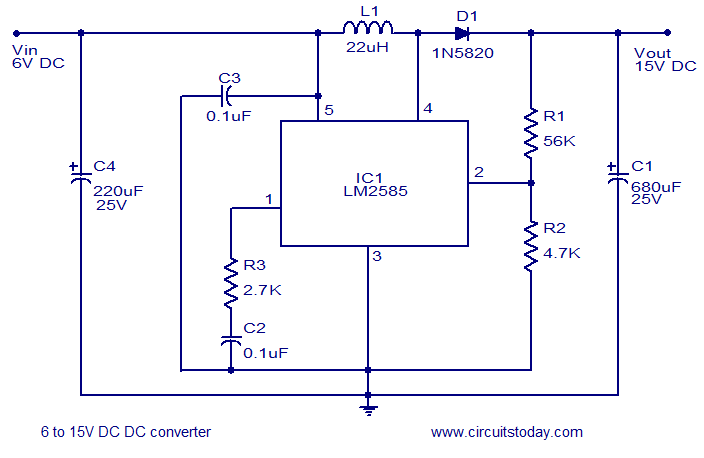## Audio

[Audio][slideshow]

## 6 to 15V DC to DC converter using LM2585

Description.
A very efficient 6V to 15V DC to DC converter using LM2585 is shown here. LM2585 is a monolithic integrated voltage converter IC that can be used in various applications like flyback converters, boost converters, forward converters, multiple output converters etc. The circuit requires minimum number of external components and the IC can source up to 3A output current.
Here the IC is wired as a boost converter where resistors R1 and R2 are used to set the output voltage .The junction of R1 and R2 is connected to the feedback pin of IC1. Capacitor C4 is the input filter while capacitor C1 the filter for output. Network comprising of resistor R1 and capacitor C2 is meant for frequency compensation. Inductor L1 stores the energy for acquiring boost conversion.

Circuit diagram of LM2585 DC to DC converter.6 to 15 V converter using LM2585

Notes.

• Assemble the circuit on a good quality PCB.
• LM2585 requires a heatsink.
• Output voltage is according to the equation Vout =( (R1/R2)+1) x 1.23.
• Capacitors other than C4 and C1 are ceramic capacitors.
• Maximum output current LM2585 can source is 3A.

[Lights][grids]